# C语言操作符与表达式小结

2018-03-25 20:56 10 查看

## 目录

c语言操作符和表达式小结

### 算数操作符

C语言中算数操作符包括：

`+ - * / %`

### 位移操作符

C语言中位移操作符包括：

`右移操作符 >> 左移操作符 <<`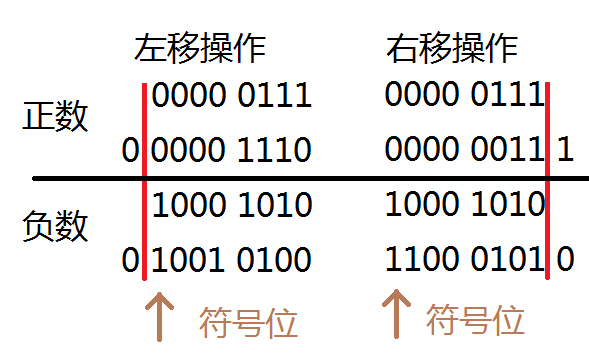*使用位移运算符时，向右移动n位，相当于除以 2^n；向左移动n位，相当于乘以2^n。

*位移运算比算数运算更加高效。

*在使用位移运算符时，通常不移动负数，例如：a<<-5。因为编译器没有对这类操作有详细的定义，在使用中应该避免这样用。

### 位操作符

`按位与 &   按位或 |  按位异或 ^`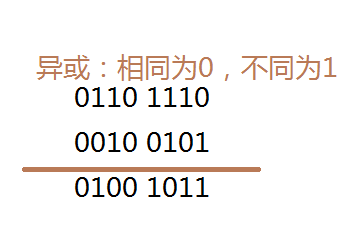### 小练习题

```#include<stdio.h>
#include<process.h>
int main()
{
int value = 0;
int count = 0;
scanf_s("%d", &value);
while (value != 0)
{
if (value < 0)
{
value = -value;
count = count + 1;// 负数的符号位加一
}
if ((value % 2) != 0)// 模2就相当于二进制表达剪掉一位
{
count = count + 1;
}
value = value >> 1;//向右位移一位
}
printf("这个数有%d个1\n", count);
system("pause");
return 0;
}```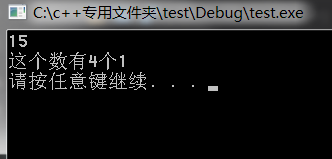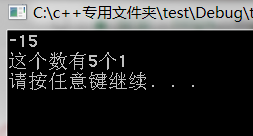### 复合运算符

`+=  -=  *=  /=  %=  <<=  >>=  &=  ^=  !=`

### 单目操作符

`！  ++   -（负号）   &   sizeof  ~  --  +（正号） *(指针）  （类型）`

* 这里主要说说sizeof，sizeof可以用来判断变量和类型的大小，它的单位是字节（byte）。

*（类型）操作符被称之为强制类型转换。如(float)a，这个操作符有很高的优先级，在对表达式使用的时候要用括号括起来，以免产生错误。

* 自增运算符需要注意的问题，++i，表示先加后用， i++，表示先用后加。

```#include<stdio.h>
#include<process.h>
int main()
{
int i = 0, a = 0, b = 0;
a = i++;
b = ++i;
printf("a = %d, b = %d\n", a, b);
system("pause");
return 0;
}```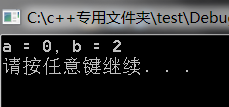### 关系操作符

`>  >=  <  <=  !=  ==`

if ( 5 == x) 数字在前，变量在后。

`&&  ||`

### 小练习题

```#include<stdio.h>
#include<process.h>
int main()
{
int i = 0, a = 0, b = 2, c = 3, d = 4;
i = a++&&++b&&d++;
//i = a++||++b||d++;
printf(" a = %d\n b = %d\n c = %d\n d = %d\n", a, b, c, d);
system("pause");
return 0;
}```0&&d++还是假，程序结束。根据短路求值，只有a++执行了操作，b和d都没有执行，所以结果如图所示。请读者朋友自行换掉注释来算得答案。

### 条件操作符

`?  :`

```if(a>5)
c[2*b + e(d/5)] = 1;
else
c[2*b + e(d/5)] = 2;
||
c[2*b + e(d/5)] = a > 5 ? 1 : 2;```

### 逗号操作符

`代码1 , 代码 2 ,……, 代码 n`

```a = f1(x);
b = f2(x + a);
for (c = f3(a, b); c > 0; c = f3(a, b))
{
a = f1(++x);
b = f2(x + a);
}
||
for (c = f3(f1(x), f2(x + a);); c > 0; c = f3(f1(++x), f2(x + a)))```

### 小练习题

```#include<stdio.h>
#include<process.h>
int main()
{
int i = 0, j = 0;
for (i = 0, j = 0; j = 0; i++, j++)
{
printf("hehe\n");
j++;
}
system("pause");
return 0;
}```

### 表达式求值

1.整形提升

```char a, b, c;
a = b
9f17
+ c;// b,c先被提升为普通整形，然后将计算结果截断，再传给a.```

2.优先级顺序

### 小练习题

```#include<stdio.h>
#include<process.h>
int fun()
{
static int count = 1;
return ++count;
}
int main()
{

int a;
a = fun() - fun()*fun();
printf("%d\n", a);
system("pause");
return 0;
}```

PS：第一个小练习题已修改，原题不能处理负数。